Most Affordable JEE | NEET | 8,9,10 Preparation by Kota's Top IITian Doctor Faculties

# NCERT Solutions for Class 9 Maths chapter 10 Exercise 10.4 - Circles - Free PDF Download

Class 9Hey, are you a class 9 Student and Looking for Ways to Download NCERT Solutions for Class 9 Maths chapter 10 Exercise 10.4? If Yes then you are at the right place.

Here we have listed Class 9 maths chapter 10 exercise 10.4 solutions in PDF that is prepared by Kota’s top IITian’s Faculties by keeping Simplicity in mind.

If you want to score high in your class 9 Maths Exam then it is very important for you to have a good knowledge of all the important topics, so to learn and practice those topics you can use eSaral NCERT Solutions.

So, without wasting more time Let’s start.

### Download The PDF of NCERT Solutions for Class 9 Maths chapter 10 Exercise 10.4 "Circles"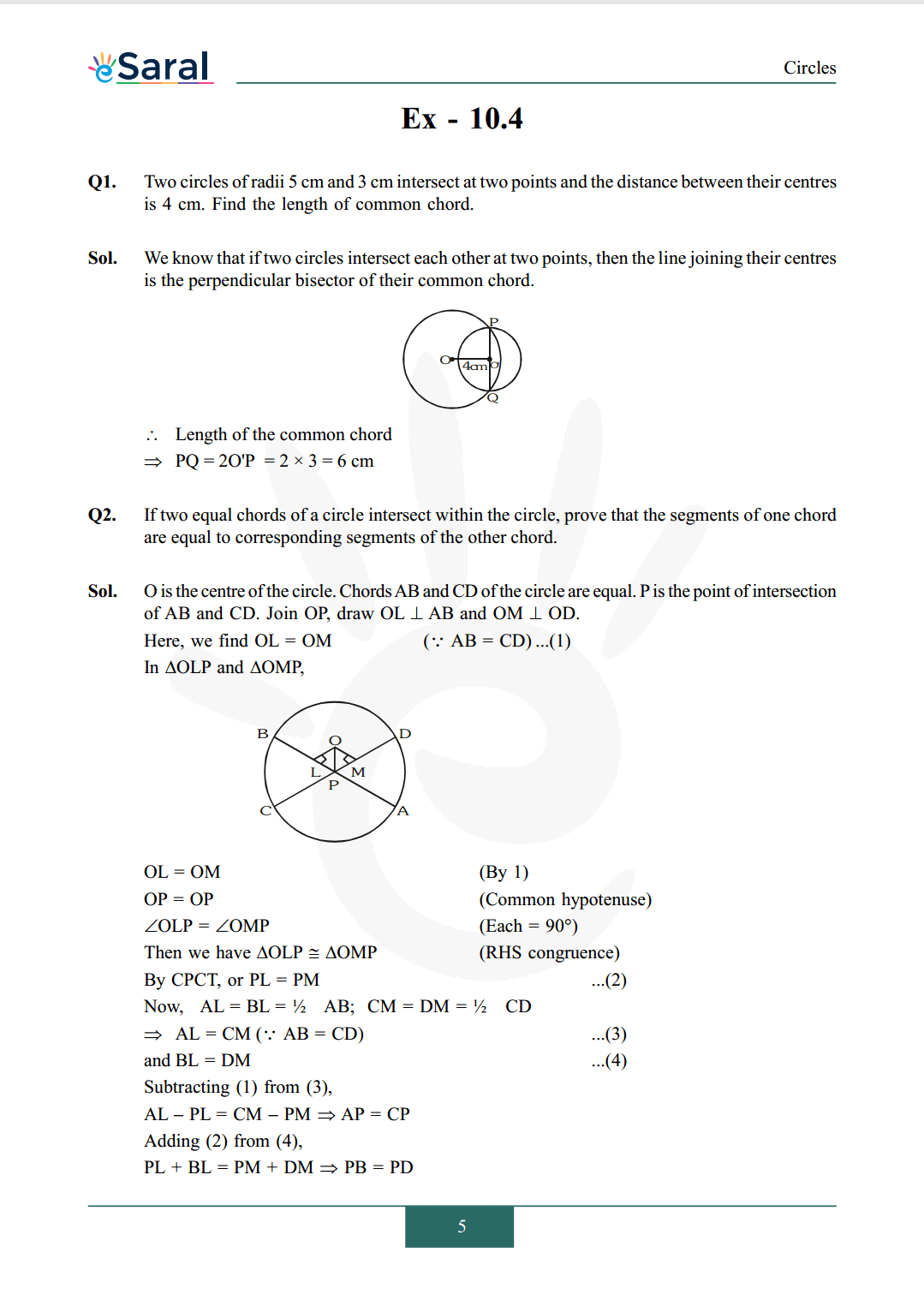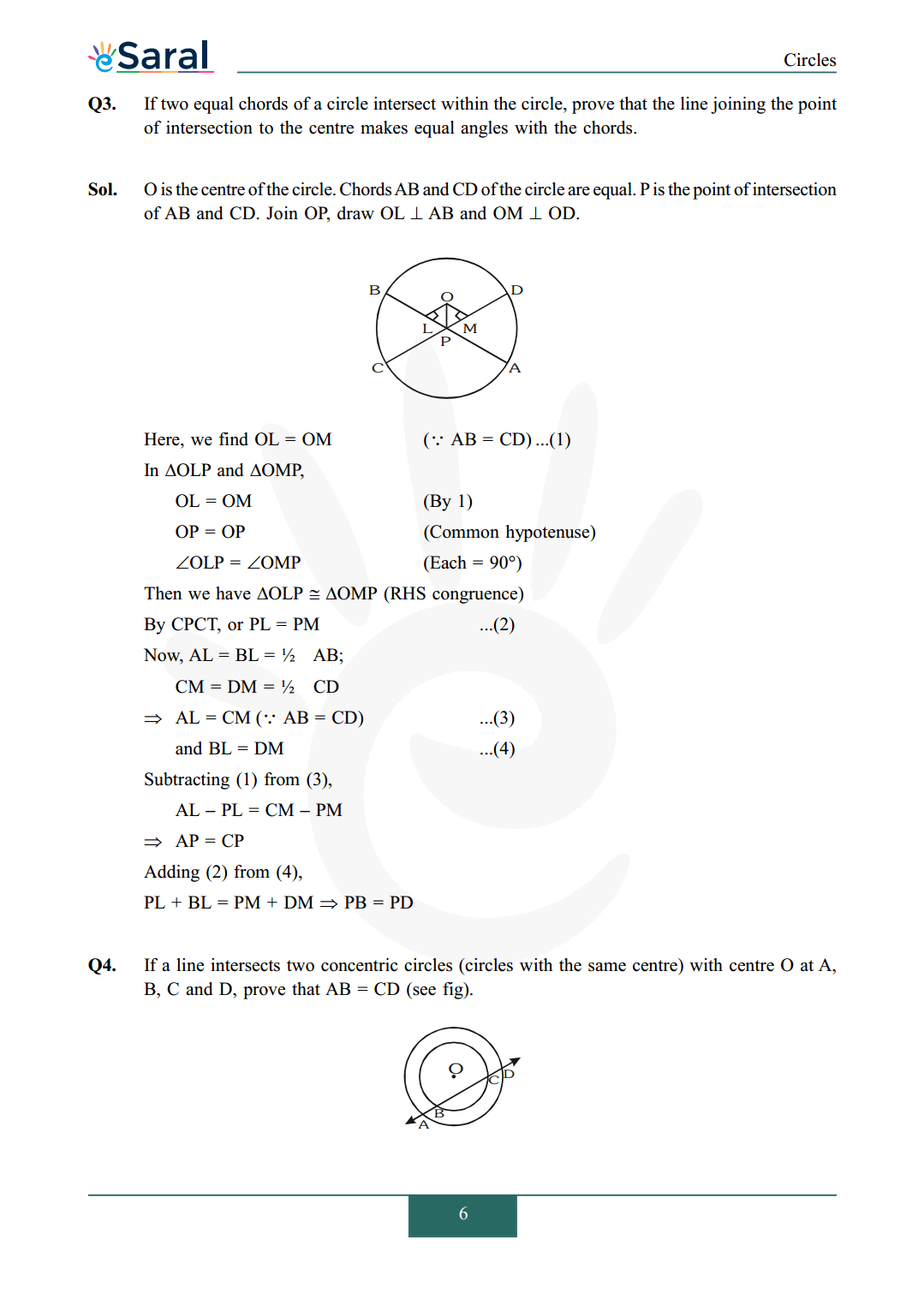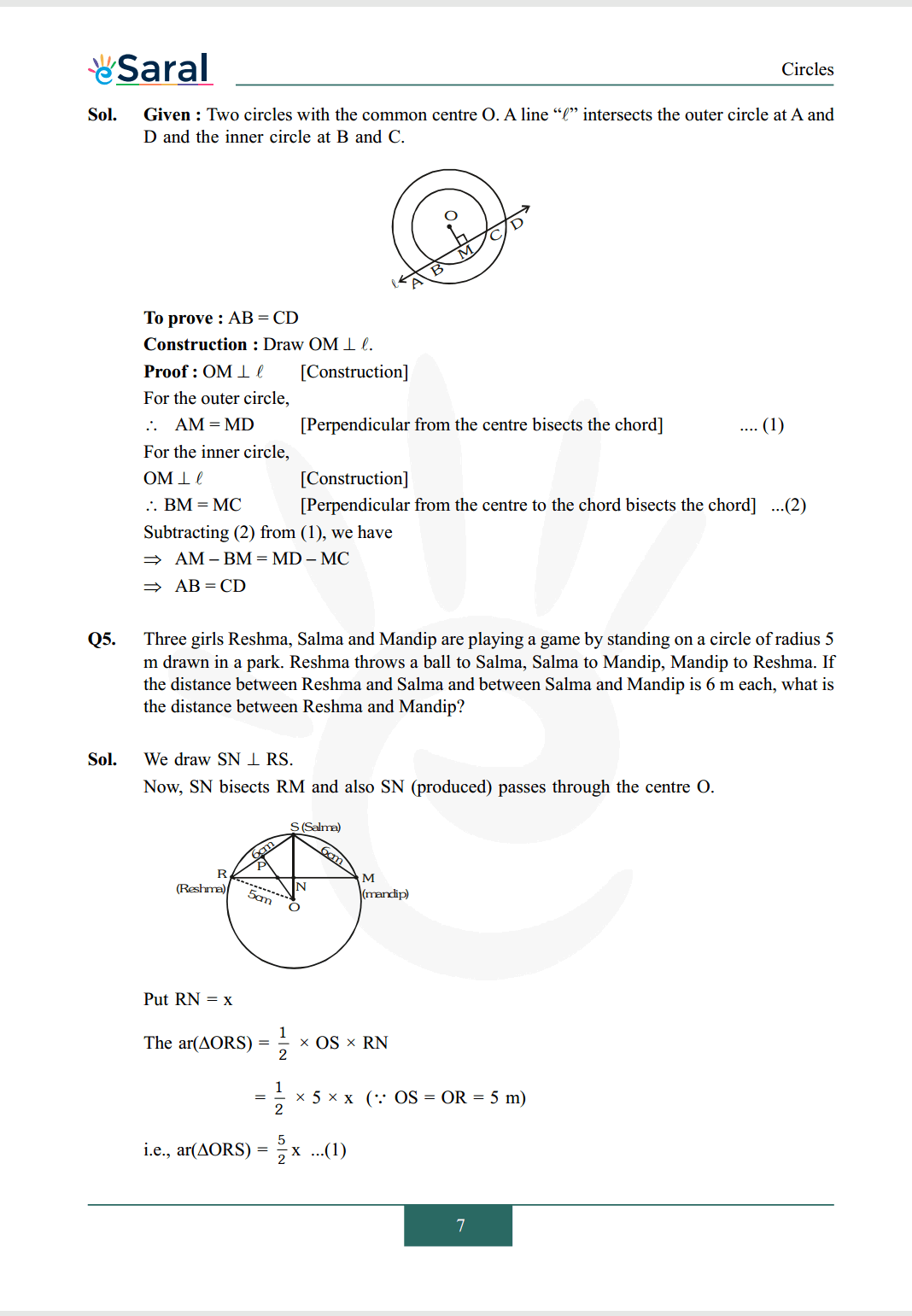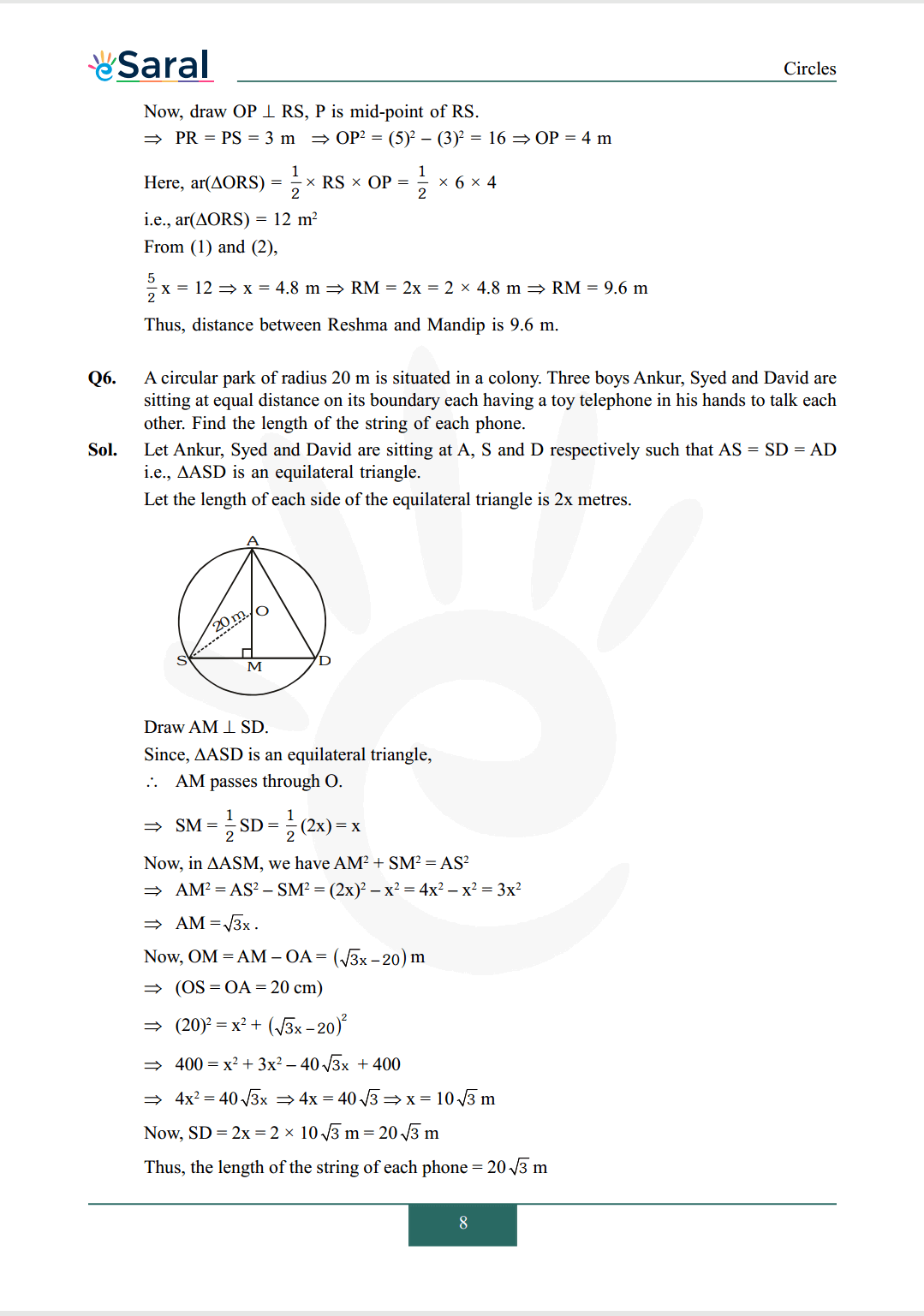All Questions of Chapter 10 Exercise 10.4

Once you complete the chapter 10 then you can revise Ex. 10.4 by solving following questions

Q1. Two circles of radii 5 cm and 3 cm intersect at two points and the distance between their centres is 4 cm. Find the length of common chord.

Q2. If two equal chords of a circle intersect within the circle, prove that the segments of one chord are equal to corresponding segments of the other chord.

Q3. If two equal chords of a circle intersect within the circle, prove that the line joining the point of intersection to the centre makes equal angles with the chords.

Q4. If a line intersects two concentric circles (circles with the same centre) with centre $\mathrm{O}$ at $\mathrm{A}$, $\mathrm{B}, \mathrm{C}$ and $\mathrm{D}$, prove that $\mathrm{AB}=\mathrm{CD}$ (see fig).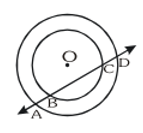Q5. Three girls Reshma, Salma and Mandip are playing a game by standing on a circle of radius 5 m drawn in a park. Reshma throws a ball to Salma, Salma to Mandip, Mandip to Reshma. If the distance between Reshma and Salma and between Salma and Mandip is 6 m each, what is the distance between Reshma and Mandip?

Q6. A circular park of radius 20 m is situated in a colony. Three boys Ankur, Syed and David are sitting at equal distance on its boundary each having a toy telephone in his hands to talk each other. Find the length of the string of each phone.